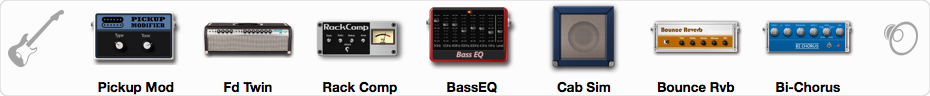# Clean

Discussion in 'ToneLib-GFX presets' started by Pietro, Feb 2, 2020.

1. Clean

Preset name: 80's Studio Clean

Effects chain:Effect: "Pickup Mod" (Dynamics / Filter), active - "yes"
"Mode" = Humbacker->Single
"Tone" = 32

Effect: "Fd Twin" (Amp simulators), active - "yes"
"Gain" = 34
"Bass" = 87
"Middle" = 60
"Treble" = 36
"Presence" = 16
"Master" = 27
"Output" = 50
"Level (dB)" = 17

Effect: "Rack Comp" (Dynamics / Filter), active - "yes"
"Threshold (dB)" = 6
"Ratio" = 1
"Attack" = Slow
"Release (ms)" = 70
"Knee" = 13
"Level (dB)" = 0

Effect: "BassEQ" (Dynamics / Filter), active - "yes"
"50 Hz" = 4
"120 Hz" = 0
"400 Hz" = -1
"500 Hz" = -2
"800 Hz" = -4
"4.5 kHz" = -2
"10 kHz" = 2
"Level (dB)" = 0

Effect: "Cab Sim" (Cabinets), active - "yes"
"Model" = 2x12" Silver Bell
"Level (dB)" = 6

Effect: "Bounce Rvb" (Reverb), active - "yes"
"Time" = 8.6
"PreDelay" = 71
"LoDamp" = 24
"HiDamp" = 42
"Mix" = 67

Effect: "Bi-Chorus" (Modulation / Sfx), active - "yes"
"Speed A" = 3.2
"Speed B" = 4.0
"Depth" = 43
"Reso" = 0
"Mode" = Parallel
"Mix" = 67

Note: You will need to download and install the ToneLib-GFX software to use the preset.

#### Attached Files:

• ###### Clean.tlgfx
File size:
617 bytes
Views:
14,281
2. IT´S PERFECT, THANKS. I LOVE THIS SOUND.